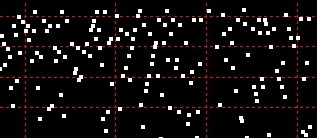NATURAL NUMBERS dedicated to explicating prime numbers without imaginary numbershome Download Proximate prime polynomial analysis up to 100,000 TXT (131KB) XLS (61KB - zip)

Perfect Prime Polynomials

Euler's Formula

Leonhard Euler's quadratic formula belongs to a select group of polynomials that should be called "Perfect Prime Polynomials". In fact, it is the best specimen of this group. The third coefficient, 41, the constant, is equal to the number of prime numbers generated without interruption (from terms 0 to 40). The only other polynomials I know of that belong to this group are n^2 - n + 3,  n^2 - n + 5 , n^2 - n + 11, and n^2 - n + 17. Both n^2 - n + 17 and n^2 - n + 41 are also proximate prime polynomials.

 Proximate Primes Polynomial Number of Primes Percent of Primes First 10 Primes Perfect Squares Finite Difference Roots 41, 43, 47, 53 n^2 - n + 41 582 58.20% 41, 43, 47, 53, 61, 71, 83, 97, 113, 131 1681 2 0.5+/-6.3835726674i

Proximate Prime Polynomials that produce more primes than Euler's formula*
* Comparison based on first 1,000 terms.

A promixate prime polynomial is a finite polynomial that is derived from four successive (proximate or neighboring) primes. The following are all quadratic (2nd degree polynomials) - that is, the first coefficient is n^2.

 53089, 53093, 53101, 53113 2n^2 - 2n + 53089 634 63.40% 53089, 53093, 53101, 53113, 53129, 53149, 53173, 53201, 53233, 53269 54289, 66049, 201601, 361201, 635209, 1194649 4 0.5+/-162.9240620657i 55661, 55663, 55667, 55673 n^2 - n + 55661 * 622 62.20% 55661, 55663, 55667, 55673, 55681, 55691, 55703, 55717, 55733, 55751 none 2 0.5+/-235.9253059763i 55663, 55667, 55673, 55681 n^2 + n + 55661 * 621 62.10% 55663, 55667, 55673, 55681, 55691, 55703, 55717, 55733, 55751, 55771 none 2 -0.5+/-235.9253059763i 55667, 55673, 55681, 55691 n^2 + 3n + 55663 * 620 62.00% 55667, 55673, 55681, 55691, 55703, 55717, 55733, 55751, 55771, 55793 none 2 -1.5+/-235.9253059763i 27941, 27943, 27947, 27953 n^2 - n + 27941 † 599 59.90% 27941, 27943, 27947, 27953, 27961, 27971, 27983, 27997, 28013, 28031 160801 2 0.5+/-167.1548683108i 27943, 27947, 27953, 27961 n^2 + n + 27941 † 599 59.90% 27943, 27947, 27953, 27961, 27971, 27983, 27997, 28013, 28031, 28051 160801 2 -0.5+/-167.1548683108i 83399, 83401, 83407, 83417 2n^2 - 4n + 83401 599 59.90% 83399, 83401, 83407, 83417, 83431, 83449, 83471, 83497, 83527, 83561 85849, 546121, 1038361 4 1+/-204.2045543077i 42701, 42703, 42709, 42719 2n^2 - 4n + 42703 593 59.30% 42701, 42703, 42709, 42719, 42733, 42751, 42773, 42799, 42829, 42863 none 4 1+/-146.1181029168i 23669, 23671, 23677, 23687 2n^2 - 4n + 23671 586 58.60% 23669, 23671, 23677, 23687, 23701, 23719, 23741, 23767, 23797, 23831 none 4 1+/-108.7864881316i

* These 3 polynomials are equivalent.
† These 2 polynomials are equivalent.